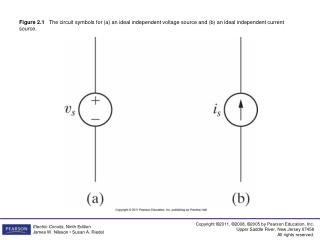DownloadDownload PresentationFigure 2.3 The circuits for Example 2.1.

# Figure 2.3 The circuits for Example 2.1.

Download Presentation## Figure 2.3 The circuits for Example 2.1.

- - - - - - - - - - - - - - - - - - - - - - - - - - - E N D - - - - - - - - - - - - - - - - - - - - - - - - - - -
##### Presentation Transcript

1. Figure 2.1 The circuit symbols for (a) an ideal independent voltage source and (b) an ideal independent current source.

2. Figure 2.2 The circuit symbols for (a) an ideal dependent voltage-controlled voltage source, (b) an ideal dependent current-controlled voltage source, (c) an ideal dependent voltage-controlled current source, and (d) an ideal dependent current-controlled current source.

3. Figure 2.3 The circuits for Example 2.1.

4. Figure 2.4 The circuits for Example 2.2.

5. Figure 2.6 Two possible reference choices for the current and voltage at the terminals of a resistor, and the resulting equations.

6. Figure 2.7 The circuit symbol for an 8 Ω resistor.

7. Figure 2.8 The circuits for Example 2.3.

8. Figure 2.10 Circuit symbols. (a) Short circuit. (b) Open circuit. (c) Switch.

9. Figure 2.11 The arrangement of flashlight components.

10. Figure 2.12 A circuit model for a flashlight.

11. Figure 2.13 The (a) device and (b) data for Example 2.5.

12. Figure 2.14 (a) The values of υt versus it for the device in Fig. 2.13. (b) The circuit model for the device in Fig. 2.13.

13. Figure 2.15 Circuit model of the flashlight with assigned voltage and current variables.

14. Figure 2.16 The circuit for Example 2.6.

15. Figure 2.17 The circuit for Example 2.7.

16. Figure 2.18 The circuit for Example 2.8.

17. Figure 2.19 The circuit shown in Fig. 2.18, with the unknowns i1, υo, and υ1 defined.

18. Figure 2.20 (a) Device and (b) data for Example 2.9.

19. Figure 2.21 (a) The graph of υt versus it for the device in Fig. 2.20(a). (b) The resulting circuit model for the device in Fig. 2.20(a), connected to a 10 Ω resistor.

20. Figure 2.22 A circuit with a dependent source.

21. Figure 2.23 The circuit for Example 2.10.

22. Figure 2.24 The circuit for Example 2.11.

23. Figure 2.25 (a) A human body with a voltage difference between one arm and one leg. (b) A simplified model of the human body with a voltage difference between one arm and one leg.

24. Figure P2.1

25. Figure P2.2

26. Figure P2.3

27. Figure P2.4

28. Figure P2.5

29. Figure P2.6

30. Figure P2.7

31. Figure P2.8

32. Figure P2.9

33. Figure P2.10

34. Figure P2.11

35. Figure P2.12

36. Figure P2.13

37. Figure P2.14

38. Figure P2.15

39. Figure P2.16

40. Figure P2.17

41. Figure P2.18

42. Figure P2.19

43. Figure P2.20

44. Figure P2.21

45. Figure P2.22

46. Figure P2.23

47. Figure P2.24